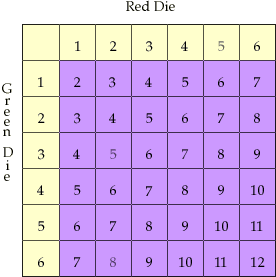Hi! My name is Carmen! I am a member of a grade 5 class in Edmonton, AB. We were talking about probability in math, and I was wanted to know what the probability (odds) of rolling two sixes would be rolling two dice? If it is not to difficult could you tell me what the probability (odds) of rolling any number with two dice were? Thank You! Sincerely, Carmen Hi Carmen, First of all we will find how many possible outcomes we have rolling two dice. Assume that the first one is Green, the second is Red. Then all possible outcomes can be represented in the following table. The numbers in the top row are the possibilities for the red die and the numbers in the first column are the possibilities for the green die. The numbers on the purple background are the sum of the numbers on the red and green dice.You can see that we have 36 possible outcomes. We are interested only in ONE outcome, a 6 on the red die and a 6 on the green die. This gives a total of 12. Thus only one of the 36 possible outcomes has a 6 on both dice and hence the probability of two sixes will be 1 over 36, or 1/36. Odds in favour 1 to 35, where 35 = 36-1. For your second question you can read the probabilities off the table. For example suppose you want "the probability of rolling a 4", that is the probability that the sum on the two dice is 4. From the table, there are 3 of the possible 36 outcomes that have a sum of 4. Hence the probability of rolling a 3 is 3/36 = 1/12. Cheers, Andrei and Penny Go to Math Central